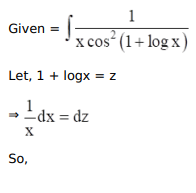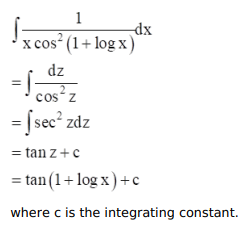# Mark against the correct answer in each of the following:

Question:

Mark $(\sqrt{)}$ against the correct answer in each of the following:

$\int \frac{1}{x \cos ^{2}(1+\log x)} d x=?$

A. $\tan (1+\log x)+C$

B. $\cot (1+\log x)+C$

C. $\sec (1+\log x)+C$

D. none of these

Solution: